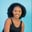Related Tags

python3

# How to use the np.mod() function for a 2D array in PythonMaria Elijah

## Overview

In Python’s NumPy library, the mod() method returns the remainder of a division.

The numpy.mod() method returns the remainder after dividing two input arrays. This is done element-wise (element by element).

Note: In Python, a list of lists can be used to create a two-dimensional (2D) array.

### Syntax

numpy.mod(x1, x2, dtype=None, out=None)


### Parameters

• x1: This is an array that represents the data input. This is the dividend.
• x2: This is an array that represents the data input. This is the divisor.
• dtype: This is an optional parameter. It represents the array’s return type.
• out: This is an optional parameter. It denotes the alternate output array where the result will be stored.

Note: If the shapes of x1 and x2 differ, they must be able to be broadcasted to a common shape for output representation.

### Return value

The numpy.mod() method returns an array that contains the remainder from the (x1/x2) arrays.

Note: In Python, the mod function is equivalent to the modulus operator, x1 % x2.

### Code

The following code shows how to use the numpy.mod() method for two-dimensional (2D) arrays:

# import numpy
import numpy as np
# create a two 2D arrays
x1 = np.array([[2,6,5],[3,4,8]])
x2 = np.array([[1,7,2],[10,9,4]])

# divide the 2D arrays
# and store the remainder in result
result = np.mod(x1, x2)

print(result)
An example of the numpy.mod() method

### Explanation

• Line 2: We import the numpy library.
• Line 4-5: We create two 2D arrays called x1 and x2.
• Line 9: We use the np.mod() method. This returns an array containing the remainder of dividing array x1 by array x2. The result is stored in a new array called result.
• Line 11: We display the result.

RELATED TAGS

python3

CONTRIBUTORMaria Elijah
RELATED COURSES

View all Courses

Keep Exploring

Learn in-demand tech skills in half the time